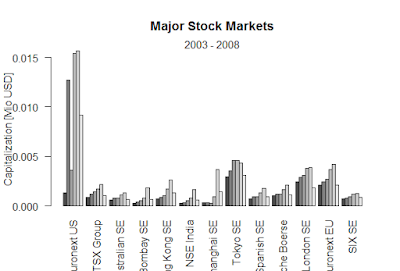Showing posts with label correlated Wiener processes are important for Analyst. Show all posts
Showing posts with label correlated Wiener processes are important for Analyst. Show all posts

## Correlated Wiener processes are important for Analyst

> library (Quantmod)
Error in library(Quantmod) : there is no package called ‘Quantmod’
> library (Quantmod)
Error in library(Quantmod) : there is no package called ‘Quantmod’
> library("quantmod", lib.loc="~/R/win-library/3.5")

Attaching package: ‘zoo’

The following objects are masked from ‘package:base’:

as.Date, as.Date.numeric

Version 0.4-0 included new data defaults. See ?getSymbols.
Learn from a quantmod author: https://www.datacamp.com/courses/importing-and-managing-financial-data-in-r
Warning messages:
1: package ‘quantmod’ was built under R version 3.5.2
2: package ‘xts’ was built under R version 3.5.1
3: package ‘zoo’ was built under R version 3.5.1
4: package ‘TTR’ was built under R version 3.5.1
> library (quantmod)
> set.seed(20140623)
> N <- 1000
> x <- cumsum(rnorm(N))
> gamma <- 0.7
> y <- gamma * x + rnorm(N)
> plot(x, type='l')
> lines(y,col="red")
> lines(y,col="green")
> z = y - gamma*x
> plot(z,type='l')
> plot(z,col=9,type='l')
> getSymbols('DTB3', src='FRED')
‘getSymbols’ currently uses auto.assign=TRUE by default, but will
use auto.assign=FALSE in 0.5-0. You will still be able to use
and getOption("getSymbols.auto.assign") will still be checked for
alternate defaults.

This message is shown once per session and may be disabled by setting
options("getSymbols.warning4.0"=FALSE). See ?getSymbols for details.

 "DTB3"
> getSymbols('DTB6', src='FRED')
 "DTB6"
> DTB3.sub = DTB3['1984-01-02/2014-03-31']
> DTB6.sub = DTB6['1984-01-02/2014-03-31']
> plot(DTB3.sub)
> lines(DTB6.sub, col='red')
> x1=as.numeric(na.omit(DTB3.sub))
> x2=as.numeric(na.omit(DTB6.sub))
> y = cbind(x1,x2)
> cregr <- lm(x1 ~ x2)
> r = cregr\$residuals
> po.coint <- po.test(y, demean = TRUE, lshort = TRUE)
Error in po.test(y, demean = TRUE, lshort = TRUE) :
could not find function "po.test"
> getSymbols("SNP", from="2004-01-01", to=Sys.Date())

WARNING: There have been significant changes to Yahoo Finance data.
Please see the Warning section of ‘?getSymbols.yahoo’ for details.

This message is shown once per session and may be disabled by setting
options("getSymbols.yahoo.warning"=FALSE).
 "SNP"
> chartSeries(Cl(SNP))
> ret <- dailyReturn(Cl(SNP), type='log')
> par(mfrow = c(2, 5))
> for (i in 1:10) {matplot(cbind(AR_result[, i], turnover_data[521:546,
+                                                              i]), type = "l", main = colnames(turnover_data)[i], xlab = "", ylab = "",
+                          col = c("red", "black"))}
Error in cbind(AR_result[, i], turnover_data[521:546, i]) :
> library("ggplot2", lib.loc="~/R/win-library/3.5")
Warning message:
package ‘ggplot2’ was built under R version 3.5.1
> install.packages("2D")
(as ‘lib’ is unspecified)
Warning in install.packages :
package ‘2D’ is not available (for R version 3.5.0)
> library("plot3D", lib.loc="~/R/win-library/3.5")
Warning message:
package ‘plot3D’ was built under R version 3.5.2
> library(plot3D)
> D2_Wiener <- function() {
+     dev.new(width = 10, height = 4)
+     par(mfrow = c(1, 3), oma = c(0, 0, 2, 0))
+     for(i in 1:3) {
+         W1 <- cumsum(rnorm(100000))
+         W2 <- cumsum(rnorm(100000))
+         plot(W1,W2, type= "l", ylab = "", xlab = "")
+     }
+     mtext("2-dimensional Wiener-processes with no correlation",
+           outer = TRUE, cex = 1.5, line = -1)
+ }
> D2_Wiener()
> Correlated_Wiener <- function(cor) {
+     dev.new(width = 10, height = 4)
+     par(mfrow = c(1, 3), oma = c(0, 0, 2, 0))
+     for(i in 1:3) {
+         W1 <- cumsum(rnorm(100000))
+         W2 <- cumsum(rnorm(100000))
+         W3 <- cor * W1 + sqrt(1 - cor^2) * W2
+         plot(W1, W3, type= "l", ylab = "", xlab = "")
+     }
+     mtext(paste("2-dimensional Wiener-processes (",cor," correlation)",
+                 sep = ""), outer = TRUE, cex = 1.5, line = -1)
+ }
> Correlated_Wiener(0.6)

### Black-Scholes formula-R

Black-Scholes formula-R > BlackScholes <- function(TypeFlag = c("c", "p"), S, X, Time, r, b, sigma) { TypeFla...